Anzeige

# Using a mobile phone as a therapist - Superweek 2018

7. Feb 2018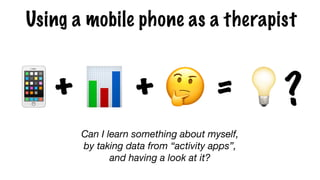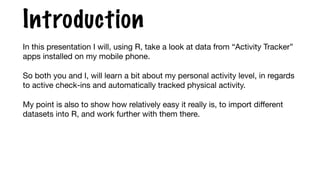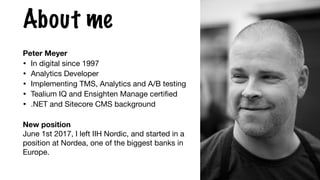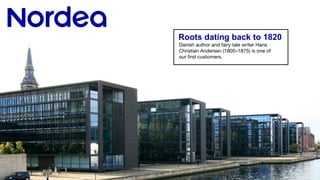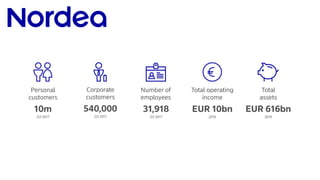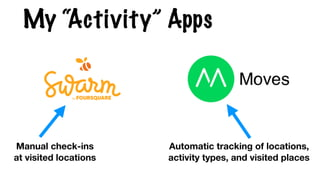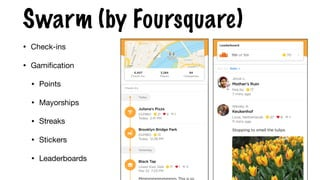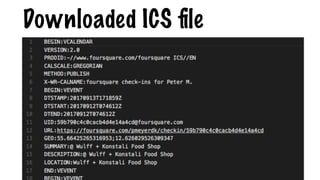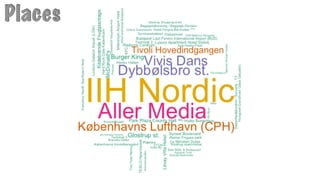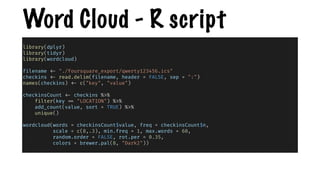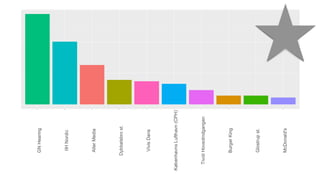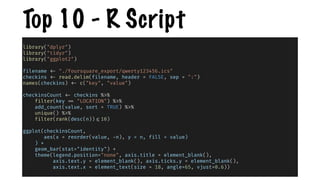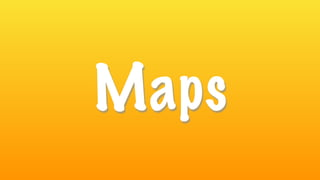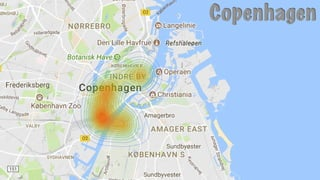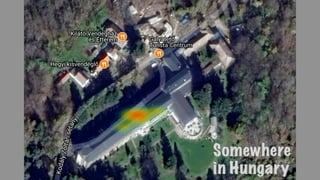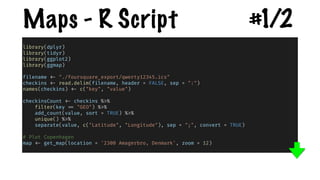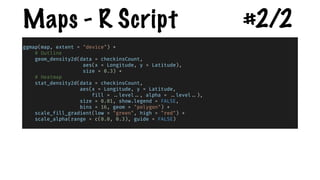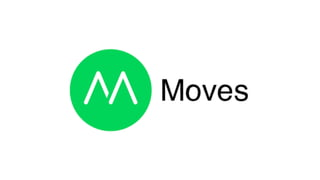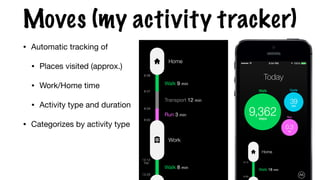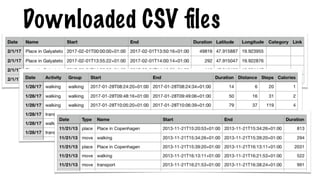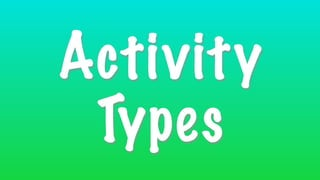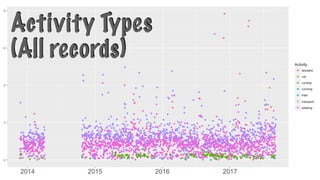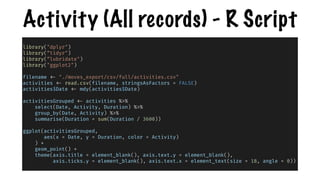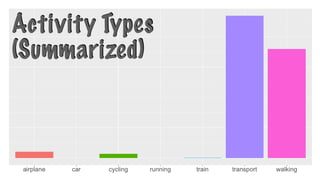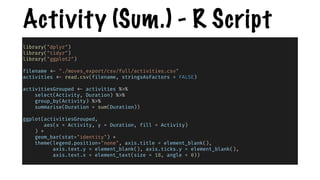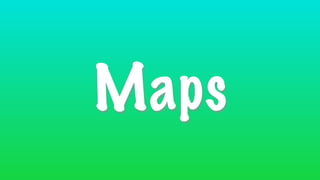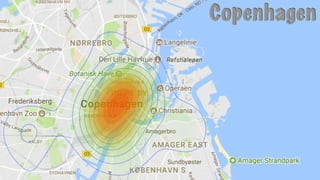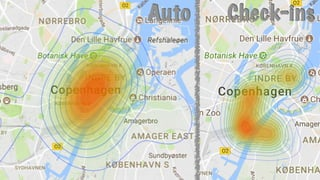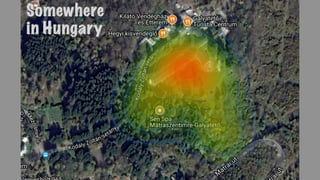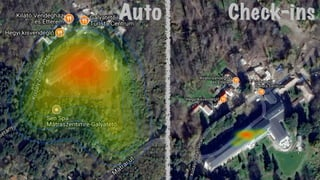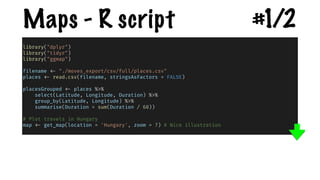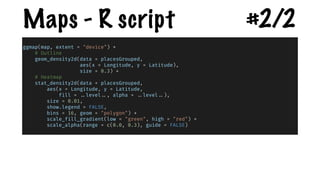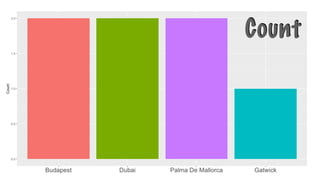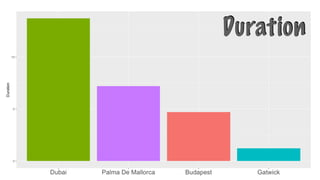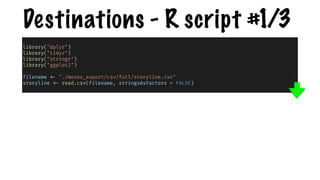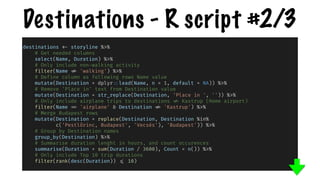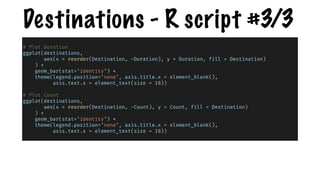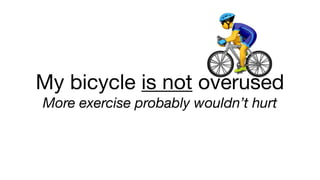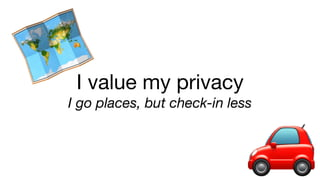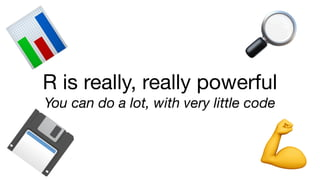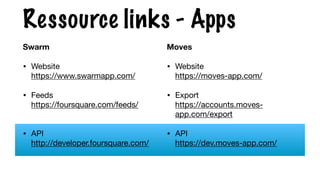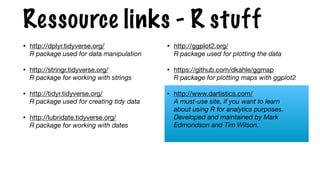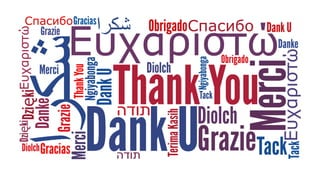1 von 56
Anzeige

### Using a mobile phone as a therapist - Superweek 2018

1. Using a mobile phone as a therapist
2. Introduction
3. 📱+ 📊 + 🤔 = 💡? Using a mobile phone as a therapist Can I learn something about myself, by taking data from “activity apps”, and having a look at it?
4. Introduction In this presentation I will, using R, take a look at data from “Activity Tracker” apps installed on my mobile phone. So both you and I, will learn a bit about my personal activity level, in regards to active check-ins and automatically tracked physical activity. My point is also to show how relatively easy it really is, to import diﬀerent datasets into R, and work further with them there.
5. About me Peter Meyer • In digital since 1997 • Analytics Developer • Implementing TMS, Analytics and A/B testing • Tealium IQ and Ensighten Manage certified • .NET and Sitecore CMS background New position  June 1st 2017, I left IIH Nordic, and started in a position at Nordea, one of the biggest banks in Europe.
6. Roots dating back to 1820 Danish author and fairy tale writer Hans Christian Andersen (1805–1875) is one of our ﬁrst customers.
7. The Apps
8. Vendors & Apps To name but a few…
9. My “Activity” Apps Manual check-ins  at visited locations Automatic tracking of locations,  activity types, and visited places
10. Swarm (by Foursquare) • Check-ins • Gamiﬁcation • Points • Mayorships • Streaks • Stickers • Leaderboards
12. Word Cloud
13. Places
14. Word Cloud - R script   library(dplyr) library(tidyr) library(wordcloud) filename <- "./foursquare_export/qwerty123456.ics" checkins <- read.delim(filename, header = FALSE, sep = ":") names(checkins) <- c("key", "value") checkinsCount <- checkins %>% filter(key == "LOCATION") %>% add_count(value, sort = TRUE) %>% unique() wordcloud(words = checkinsCount\$value, freq = checkinsCount\$n, scale = c(8,.3), min.freq = 1, max.words = 60, random.order = FALSE, rot.per = 0.35, colors = brewer.pal(8, "Dark2"))
15. Top 10
16. Top 10 - R Script library("dplyr") library("tidyr") library("ggplot2") filename <- "./foursquare_export/qwerty123456.ics" checkins <- read.delim(filename, header = FALSE, sep = ":") names(checkins) <- c("key", "value") checkinsCount <- checkins %>% filter(key == "LOCATION") %>% add_count(value, sort = TRUE) %>% unique() %>% filter(rank(desc(n)) <=10) ggplot(checkinsCount, aes(x = reorder(value, -n), y = n, fill = value) ) + geom_bar(stat="identity") + theme(legend.position="none", axis.title = element_blank(), axis.text.y = element_blank(), axis.ticks.y = element_blank(), axis.text.x = element_text(size = 18, angle=65, vjust=0.6))
17. Maps
18. Swarm - CopenhagenCopenhagen
19. Swarm - Hungary Somewhere  in Hungary
20. Maps - R Script #1/2 library(dplyr) library(tidyr) library(ggplot2) library(ggmap) filename <- "./foursquare_export/qwerty12345.ics" checkins <- read.delim(filename, header = FALSE, sep = ":") names(checkins) <- c("key", "value") checkinsCount <- checkins %>% filter(key == "GEO") %>% add_count(value, sort = TRUE) %>% unique() %>% separate(value, c("Latitude", "Longitude"), sep = ";", convert = TRUE) # Plot Copenhagen map <- get_map(location = '2300 Amagerbro, Denmark', zoom = 12)
21. Maps - R Script #2/2 ggmap(map, extent = "device") + # Outline geom_density2d(data = checkinsCount, aes(x = Longitude, y = Latitude), size = 0.3) + # Heatmap stat_density2d(data = checkinsCount, aes(x = Longitude, y = Latitude, fill = ..level .., alpha = ..level ..), size = 0.01, show.legend = FALSE, bins = 16, geom = "polygon") + scale_fill_gradient(low = "green", high = "red") + scale_alpha(range = c(0.0, 0.3), guide = FALSE)
22. Moves (my activity tracker) • Automatic tracking of • Places visited (approx.) • Work/Home time • Activity type and duration • Categorizes by activity type
24. Activity Types
25. Activity Types  (All records)
26. Activity (All records) - R Script library("dplyr") library(“tidyr")  library(“lubridate") library("ggplot2") filename <- "./moves_export/csv/full/activities.csv" activities <- read.csv(filename, stringsAsFactors = FALSE) activities\$Date <- mdy(activities\$Date) activitiesGrouped <- activities %>% select(Date, Activity, Duration) %>% group_by(Date, Activity) %>% summarise(Duration = sum(Duration / 3600)) ggplot(activitiesGrouped, aes(x = Date, y = Duration, color = Activity) ) + geom_point() + theme(axis.title = element_blank(), axis.text.y = element_blank(), axis.ticks.y = element_blank(), axis.text.x = element_text(size = 18, angle = 0))
27. Activity Types  (Summarized)
28. Activity (Sum.) - R Script library("dplyr") library("tidyr") library("ggplot2") filename <- "./moves_export/csv/full/activities.csv" activities <- read.csv(filename, stringsAsFactors = FALSE) activitiesGrouped <- activities %>% select(Activity, Duration) %>% group_by(Activity) %>% summarise(Duration = sum(Duration)) ggplot(activitiesGrouped, aes(x = Activity, y = Duration, fill = Activity) ) + geom_bar(stat="identity") + theme(legend.position="none", axis.title = element_blank(), axis.text.y = element_blank(), axis.ticks.y = element_blank(), axis.text.x = element_text(size = 18, angle = 0))
29. Maps
30. Copenhagen
31. Check-insAuto
32. Somewhere  in Hungary
33. Check-insAuto
34. library("dplyr") library("tidyr") library("ggmap") filename <- "./moves_export/csv/full/places.csv" places <- read.csv(filename, stringsAsFactors = FALSE) placesGrouped <- places %>% select(Latitude, Longitude, Duration) %>% group_by(Latitude, Longitude) %>% summarise(Duration = sum(Duration / 60)) # Plot travels in Hungary map <- get_map(location = 'Hungary', zoom = 7) # Nice illustration Maps - R script #1/2
35. ggmap(map, extent = "device") + # Outline geom_density2d(data = placesGrouped, aes(x = Longitude, y = Latitude), size = 0.3) + # Heatmap stat_density2d(data = placesGrouped, aes(x = Longitude, y = Latitude, fill = ..level .., alpha = ..level ..), size = 0.01, show.legend = FALSE, bins = 16, geom = "polygon") + scale_fill_gradient(low = "green", high = "red") + scale_alpha(range = c(0.0, 0.3), guide = FALSE) Maps - R script #2/2
36. Airplane Destinations
37. Count
38. Duration
39. Destinations - R script #1/3 library("dplyr") library("tidyr") library("stringr") library("ggplot2") filename <- "./moves_export/csv/full/storyline.csv" storyline <- read.csv(filename, stringsAsFactors = FALSE)
40. Destinations - R script #2/3 destinations <- storyline %>% # Get needed columns select(Name, Duration) %>% # Only include non-walking activity filter(Name != 'walking') %>% # Define column as following rows Name value mutate(Destination = dplyr ::lead(Name, n = 1, default = NA)) %>% # Remove 'Place in' text from Destination value mutate(Destination = str_replace(Destination, 'Place in ', '')) %>% # Only include airplane trips to destinations != Kastrup (Home airport) filter(Name == 'airplane' & Destination != 'Kastrup') %>% # Merge Budapest rows mutate(Destination = replace(Destination, Destination %in% c('Pestlőrinc, Budapest', 'Vecsés'), 'Budapest')) %>% # Group by Destination names group_by(Destination) %>% # Summarise duration lenght in hours, and count occurences summarise(Duration = sum(Duration / 3600), Count = n()) %>% # Only include Top 10 trip durations filter(rank(desc(Duration)) <= 10)
41. Destinations - R script #3/3 # Plot Duration ggplot(destinations, aes(x = reorder(Destination, -Duration), y = Duration, fill = Destination) ) + geom_bar(stat="identity") + theme(legend.position="none", axis.title.x = element_blank(), axis.text.x = element_text(size = 18)) # Plot Count ggplot(destinations, aes(x = reorder(Destination, -Count), y = Count, fill = Destination) ) + geom_bar(stat="identity") + theme(legend.position="none", axis.title.x = element_blank(), axis.text.x = element_text(size = 18))
42. What I learned from my therapist
43. 🚴My bicycle is not overused  More exercise probably wouldn’t hurt
44. I value my privacy  I go places, but check-in less 🗺 🚗
45. R is really, really powerful  You can do a lot, with very little code 📊 💾 🔎 💪